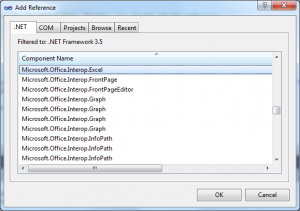11 Jul

This example shows how to read data from excel file using C#. To achive this, firstly, we need to add a reference to the dynamic link library for Excel which is called Microsoft.Office.Interop.Excel.dll. Firstly, go to your solution explorer and click on add a reference. Then, add Microsoft.Office.Interop.Excel.dll.

Also you must add the using to the following code.

using Excel = Microsoft.Office.Interop.Excel;Usage:

{
Excel.Application excelApp = new Excel.Application();
if (excelApp != null)
{
Excel.Workbook excelWorkbook = excelApp.Workbooks.Open(@"C:\test.xls", 0, true, 5, "", "", true, Excel.XlPlatform.xlWindows, "\t", false, false, 0, true, 1, 0);
Excel.Worksheet excelWorksheet = (Excel.Worksheet)excelWorkbook.Sheets;

Excel.Range excelRange = excelWorksheet.UsedRange;
int rowCount = excelRange.Rows.Count;
int colCount = excelRange.Columns.Count;

for (int i = 1; i <= rowCount; i++)
{
for (int j = 1; j <= colCount; j++)
{
Excel.Range range = (excelWorksheet.Cells[i, 1] as Excel.Range);
string cellValue = range.Value.ToString();

//do anything
}
}

excelWorkbook.Close();
excelApp.Quit();
}
}

2 thoughts on “C# Read Data From Excel File”

1.The variable J is never used. Your example is just for the first column?
Would this be the general case?
Excel.Range range = (excelWorksheet.Cells[i, j] as Excel.Range);

2.Good article, but it is dependent on Interop which need MS Office installed on Server.
If anyone don’t want to use Interop, they can use EPPlus or OleDB to read excel.
Check C# read excel file using OleDb/EPPlus